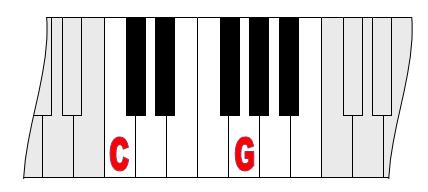#### You may also like### Archimedes and Numerical Roots

The problem is how did Archimedes calculate the lengths of the sides of the polygons which needed him to be able to calculate square roots?### Rationals Between...

What fractions can you find between the square roots of 65 and 67?### Root to Poly

Find the polynomial p(x) with integer coefficients such that one solution of the equation p(x)=0 is $1+\sqrt 2+\sqrt 3$.

# Equal Temperament

##### Age 14 to 16 Challenge Level:

You may wish to explore Problem 1 (Six Notes all Nice Ratios ) and Problem 2 (Pythagoras' Comma ) before trying this problem.The scale on a piano does something clever : the ratio (interval) between any adjacent points on the scale is equal. The keyboard is a repeating pattern of seven white keys with a black key in between two whites in five places. If you play any note, twelve points higher will be exactly an octave on.

The piano is made this way to allow a key change in the music without re-tuning the instrument.

C to G is the two to three ratio, explored in the earlier problems. It's called a "fifth", because it's the fifth note along the white keys starting from C .

Is C to G an exact two to three ratio, or just off and, if so, by how much?